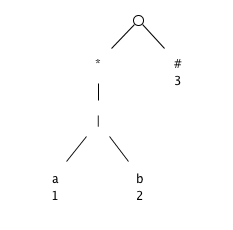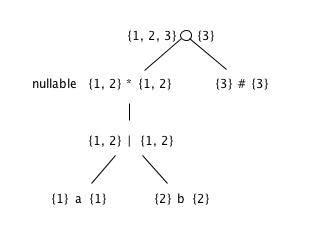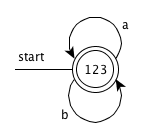• Introduction
• 1. 1.Introduction
• 2. 2.A Simple Syntax-Directed Translator
• 3. 3.Lexical Analysis
• 4. 4.Syntax Analysis
• 5. 5.Syntax-Directed Translation
• 6. 6.Intermediate-Code Generation
• 7. 7.Run-Time Environments
• 8. 8.Code Generation
• 9. 12.InterProcedural Analysis
• Published with GitBook

# Exercises for Section 3.9

### 3.9.1

Extend the table of Fig. 3.58 to include the operators

1. +

node n nullable(n) firstpos(n)
n = c_1 ? true firstpos(c_1)
n = c_1 + nullable(c_1) firstpos(c_1)

### 3.9.2

Use Algorithm 3.36 to convert the regular expressions of Ex­ercise 3.7.3 directly to deterministic finite automata.

1. (a|b)*

• Syntax tree• firstpos and lastpos for nodes in the syntax tree``````- The function followpos

<table>
<tr>
<th>node n</th>
<th>followpos(n)</th>
</tr>
<tbody>
<tr>
<td>1</td>
<td>{1, 2, 3}</td>
</tr>
<tr>
<td>2</td>
<td>{1, 2, 3}</td>
</tr>
<tr>
<td>3</td>
<td>∅</td>
</tr>
</tbody>
</table>
``````
• Steps

The value of firstpos for the root of the tree is {1, 2, 3}, so this set is the start state of D. Call this set of states A. We compute Dtran[A, a] and Dtran[A, b]. Among the positions of A, 1 correspond to a, while 2 correspond to b. Thus Dtran[A, a] = followpos(1) = {1, 2, 3}， Dtran[A, b] = followpos(2) = {1, 2, 3}. Both the results are set A, so dose not have new state, end the computation.

• DFA1. (a*|b*)*

2. ((ε|a)|b*)*

3. (a|b)*abb(a|b)*

### 3.9.3 !

We can prove that two regular expressions are equivalent by showing that their minimum-state DFA's are the same up to renaming of states. Show in this way that the following regular expressions: (a|b)*, (a*|b*)*, and ((ε|a)b*)* are all equivalent. Note: You may have constructed the DFA's for these expressions in response to Exercise 3.7.3.

Refer to the answers of 3.7.3 and 3.9.2-1

### 3.9.4 !

Construct the minimum-state DFA's for the following regular expressions:

1. (a|b)*a(a|b)
2. (a|b)*a(a|b)(a|b)
3. (a|b)*a(a|b)(a|b)(a|b)

Do you see a pattern?

### 3.9.5 !!

To make formal the informal claim of Example 3.25, show that any deterministic finite automaton for the regular expression

(a|b)*a(a|b)...(a|b)

where (a|b) appears n - 1 times at the end, must have at least 2n states. Hint: Observe the pattern in Exercise 3.9.4. What condition regarding the history of inputs does each state represent?